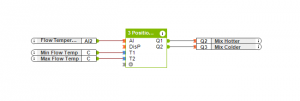# 3 Position Controller

## INFORMATION

With a 3 Position controller the two outputs Q1 and Q2 states depend on the two target values T1 and T2 and the actual analogue input value at AI. If DisP is set to be on, then all the outputs Q1 and Q2 are set to be off and the controller is disabled.

If the value at AI is less than the parameter T1 the output Q1 will be on.

If the value of AI is between the two parameters T1 and T2 then both the outputs Q1 and Q2 will be off.

If the value of AI is greater than parameter T2 then the output Q2 is activated.

#### EXAMPLEIn this example we have a 2 port mixing valve that is controlled by two digital outputs, one for moving to fully open position and one for moving to fully closed position.

If the flow temperature is below the parameter T1 which is set using a constant, the valve will move to the fully open position. If the flow temperature is higher than parameter T2 then the valve will move to fully closed position. If the flow temperature is within the range then the valve will be in a middle position.